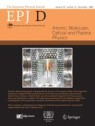# Quantum momentum

A new quantum-mechanical model has been developed that allows the momentum of quantum particles to be measured using a variant of the classical time-of-flight

New York | Heidelberg, 5 August 2019Quantum mechanics is an extraordinarily successful way of understanding the physical world at extremely small scales. Through it, a handful of rules can be used to explain the majority of experimentally observable phenomena. Occasionally, however, we come across a problem in classical mechanics that poses particular difficulties for translation into the quantum world. A new study published in EPJ D has provided some insights into one of them: momentum. The authors, theoretical physicists Fabio Di Pumpo and Matthias Freyberger from Ulm University, Germany, present an elegant mathematical model of quantum momentum that is accessible through another classical concept: time-of-flight.

Many people will recall the traditional definition of momentum from high-school physics as being the product of the mass of an object and the velocity at which it is travelling. In quantum theory an object is represented by a wave function and its position cannot be determined unless the wave function is 'collapsed' into a single state. This is the essence of measurement in quantum mechanics.

Classical momentum can be obtained simply by measuring the time an object takes to pass between two stationary detectors ('time-of-flight'), finding the velocity and multiplying by the mass. Di Pumpo and Freyberger have developed a model of the quantum equivalent of this experiment in which the roles of time and distance are reversed: the time points are fixed, and the probabilistic positions of a wave function at each point, and thus the distance between them, estimated. This approach uses additional quantum systems called pointers that are coupled to a moving wave packet using a method developed by von Neumann, with measurements made to the pointers rather than the wave.

Di Pumpo and Freyberger were thus able to derive a single, measurable quantity that is a quantum equivalent of the classical time-of-flight, and to calculate the momentum of a quantum particle quite precisely on this basis. They end the paper by suggesting ways of further improving the accuracy of the measurement.

References: F. Di Pumpo and M. Freyberger (2019) Pointer-based model for state reduction in momentum space, European Physical Journal D 73: 163, DOI: 10.1140/epjd/e2019-100226-1

Further Information